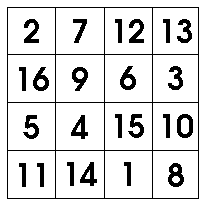Magic Squares

All about magic squares!

By

Magic squares are amazing things. If you aren't sure what a magic square is, it's a square grid of numbers containing all the numbers 1, 2, 3 and so on, each one exactly once. What's more, each row, column and diagonal of a magic square must add up to the same number! An example is shown here :Notice the sum of the rows are all the same :

• 2 + 7 + 12 + 13 = 34
• 16 + 9 + 6 + 3 = 34
• 5 + 4 + 15 + 10 = 34 as well, and
• 11 + 14 + 1 + 8 = 34!!
Also, the two diagonals each add up to 34 :
• 2 + 9 + 15 + 8 = 34!
• 11 + 4 + 6 + 13 = 34 again!!
As do the four columns!
• 2 + 16 + 5 + 11 = 34,
• 7 + 9 + 4 + 14 = 34,
• 12 + 6 + 15 + 1 = 34, and
• 13 + 3 + 10 + 8 = 34!

This number 34 is called the Magic Number of the magic square.

Magic squares have a long history, dating back to ancient China. On this site, you can find a bunch of magic square resources, including two recipes to help you make a magic square, and a magic square java applet to help you when the recipes don't work. There's also a way to combine small magic squares, to make bigger ones.

You'll also find some magic square puzzle worksheets, and instructions on how to make your own magic square puzzles.

And did you know there's an amazing link between this arithmetic game and the three by three magic square? It's true! See if you can figure out what it is. Drop me a line when you do!

Yours, Dr Mike...

If you like what you've just read, sign up for this site's free newsletters:
• Monday Morning Math: A weekly email of fascinating math facts - how math works in everyday life.
• New Game Alerts: Be first to know when I upload new games.
Get these free now! Enter Your Email: